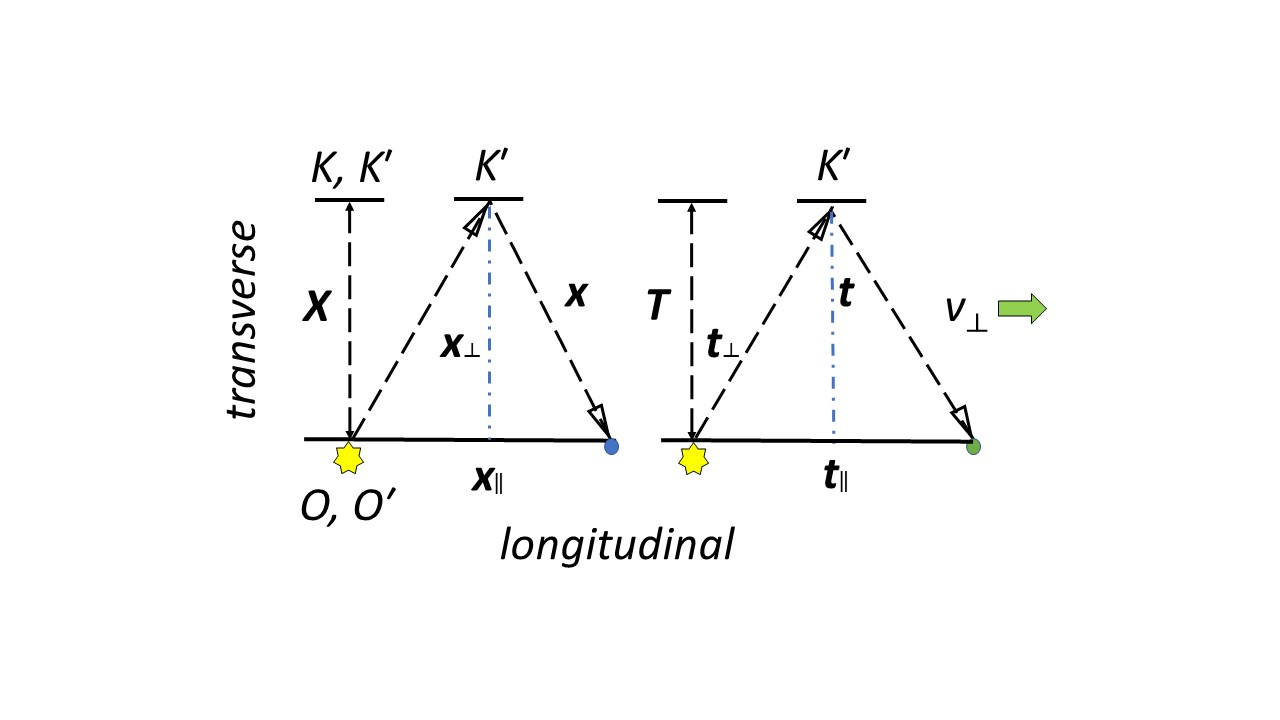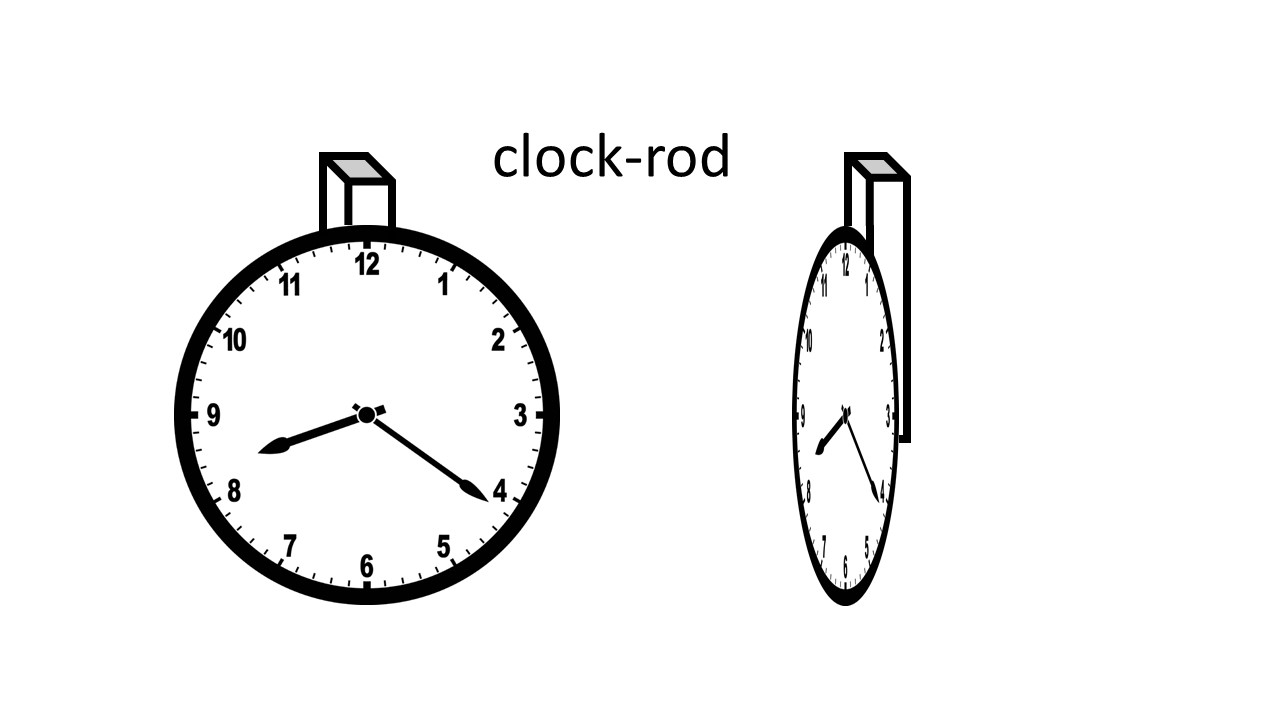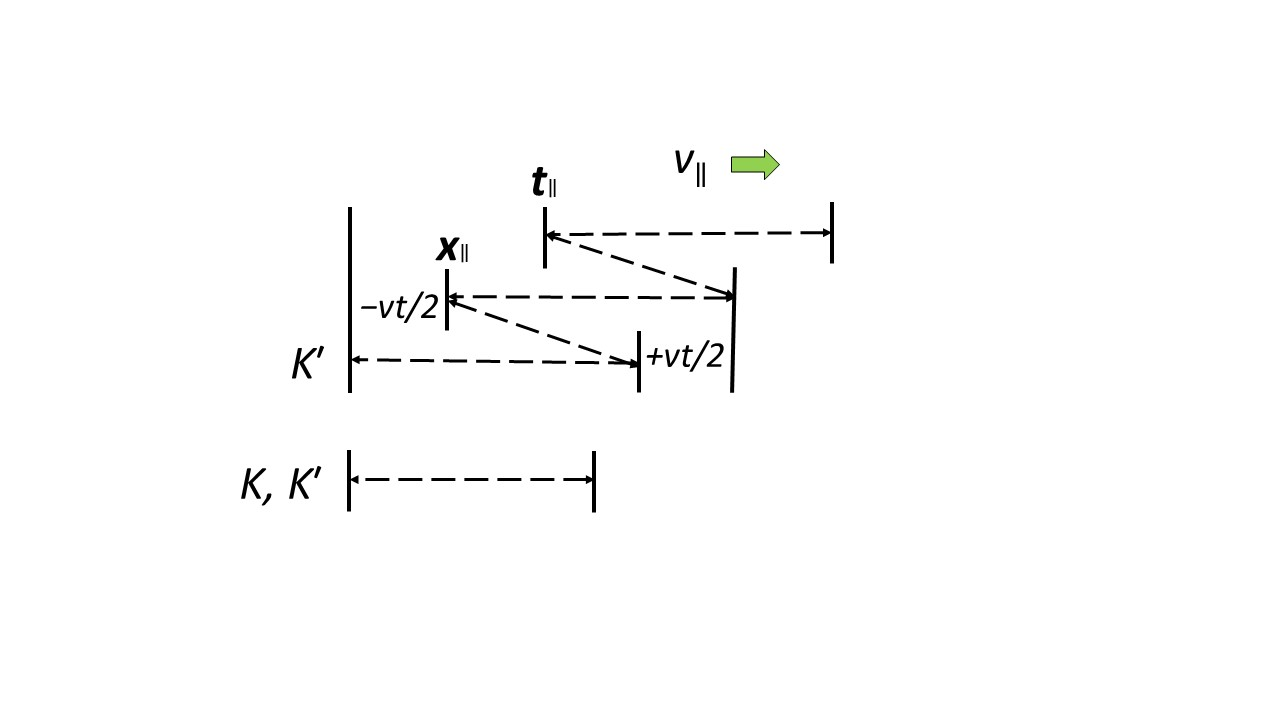iSoul In the beginning is reality.

# Category Archives: Knowing

epistemology, science, kinds of knowledge, methodology

# Harmonic algebra

This revised post follows up on harmonic addition mentioned in a previous post here.

Harmonic algebra is an inverse algebra. It is based on an automorphism that interchanges the zero with the infinite and the greater-than-one with the less-than-one: 0 ↔ ∞ and x ↔ 1/x. So zero becomes the new inaccessible number and infinity becomes the new additive unit. That is,

xy := g−1(g(x) + g(y))

xy := g−1(g(x) ∙ g(y))

etc., where g(x) = 1/x with x ≠ 0.

Regular and harmonic algebra are isomorphic with ordinary addition exchanged with harmonic multiplication and ordinary multiplication exchanged with harmonic division. Harmonic algebra is like counting down from infinity, in which an increment of one reduces the amount slightly.

Harmonic addition is defined as a power operation:

with the understanding that (1/0) → ∞ and (1/∞) → 0. An intermediate value may be zero but not a final value.

Simple harmonic addition is thus defined as:

# Lorentz in spacetime and timespace

This post builds on the previous posts here and here, and follows the approach of J.-M. Levy here.

First, consider the Lorentz transformation in spacetime along the x axis per Levy’s section III:

Let us now envision two frames in ‘standard configuration’ with having velocity v with respect to K and let x, t (resp. x´, t´) be the coordinates of event M in the two frames. Let O and be the spatial origins of the frames; O and cöıncide at time t = = 0.

Here comes the pretty argument: all we have to do is to express the relation

OM = OO´ + O´M (equation 5)

between vectors (which here reduce to oriented segments) in both frames.

In K, OM = x, OO´ = vt and O´M seen from K is /γ since is O′M as measured in . Hence a first relation is:

x = vt + /γ (equation 6)

In , OM = x/γ since x is OM as measured in K, OO′ = vt´ and O′M = . Hence a second relation:

x/γ = vt´+ x´ (equation 7)

# Light clocks with multidimensional time

A previous post on this subject is here. One reference for this post is V. A. Ugarov’s Special Theory of Relativity (Mir, 1979).

A light clock is a device with an emission-reflection-reception cycle of light that registers the current timeline point and placeline point in units of cycle length and duration. Consider two identical light clocks, at first in their reference frames at rest, K, K´. Then, as the light clock in K´ moves transversely relative to K with uniform motion at velocity v (right), from K one observes the following:The illustration above shows one cycle length of the light path (i.e., wavelength), X, on the left and one cycle duration (i.e., period), T, on the right at rest in reference frames K, K´. For the reference frame K´, in motion relative to reference frame K, call the arc length of one cycle of the light path x. Call the distance between the beginning and ending place points of one cycle x. For the reference frame K´ relative to reference frame K, call the arc time of one cycle of the light path t. Call the distime between the beginning and ending timepoints of one cycle t.

# Clock-rods

A clock-rod is a linear or planar clock with a parallel rod attached to it. A mechanical or electronic clock-rod might look like this:Three clock-rods mutually perpendicular would measure length and duration in all directions.

A light clock-rod is conceptually like this:The clock and rod are parallel to each other so that parallel or perpendicular motion would change either the measurements of either the clock or the rod but not both. A complete harmonic cycle is not affected by motion:The +vt/2 increased distance of the first half-cycle is offset by the −vt/2 decreased distance of the second half-cycle. Likewise for the times +2d/v and −2d/v.

# Kinematic proofs

Displacement with distime: displacement s, distime (time interval) t, velocities v1 and v, acceleration a:

To prove: v = v1 + at

a = (vv1) / t    by definition

at = (vv1)     multiply by t

v = v1 + at

To prove: s = v1t + ½ at2

vavg = s / t          by definition

vavg = (v1 + v) / 2        by definition

s / t = (v1 + v) / 2       combining these two

s = (v1 + v) t / 2         multiply by t

s = (v1 + (v1 + at)) t / 2       from above

s = (2v1 + at) t / 2

s = v1t + ½ at2

# Space as time and time as spaceGalileo used the distance of uniform motion as a measure of the distime, i.e., time interval (Dialogues Concerning Two New Sciences Tr. by Henry Crew and Alfonso de Salvio, 1914):

Accordingly we see that while the body moves from b to c with uniform speed, it also falls perpendicularly through the distance ci, and at the end of the time-interval bc finds itself at the point i. p.199

Without getting into the details of the Figure 108, notice the shift of language: “the body moves from b to c” [i.e., a distance], then “the distime bc“. Galileo uses a distance to measure a distime, which is justified since the motion is “with uniform speed” and so they are proportional.

The point to make here is that a distance and a distime can be interchanged if the motion is uniform. That is exactly the function of a clock: to provide a standard distime for a corresponding distance of motion. The change from distance to distime is basically a change of units. So, the line with a to e and beyond is a linear clock: it measures elapsed distime or “elapsed distance”.

Let there a ball be dropped out the window by a passenger on a train in uniform motion. Consider the following four scenarios, in which the distance or distime of a uniform motion is measured: (1) looking down above the moving ball, measuring the distance of fall; (2) looking down above the moving ball, measuring the (uniform) distime of fall; (3) looking from the side, measuring the distance of motion in two dimensions; and (4) looking from the side, measuring the (uniform) distime of motion in two dimensions.

# Metric postulates for time geometry

Geometry was developed by the ancient Greeks in the language of length, but it is an abstraction that may be applied to anything that conforms to its definitions and axioms. Here we apply it to duration. We will use Brossard’s “Metric Postulates for Space Geometry” [American Mathematical Monthly, Vol. 74, No. 7, Aug.-Sep., 1967, pp. 777-788], which generalizes the plane metric geometry of Birkhoff to three dimensions. First, Brossard:

Primitive notions. Points are abstract undefined objects. Primitive terms are: point, distance, line, ray or half-line, half-bundle of rays, and angular measure. The set of all points will be denoted by the letter S and some subsets of S are called lines. The plane as well as the three-dimensional space shall not be taken as primitive terms but will be constructed.

Axioms on points, lines, and distance. The axioms on the points and on the lines are:

E1. There exist at least two points in S.

E2. A line contains at least two points.

E3. Through two distinct points there is one and only one line.

E4. There exist points not all on the same line.

A set of points is said to be collinear if this set is a subset of a line. Two sets are collinear if the union of these sets is collinear. The axioms on distance are:

D1. If A and B are points, then d(AB) is a nonnegative real number.

D2. For points A and B, d(AB) = 0 if and only if A = B.

D3. If A and B are points, then d(AB) = d(BA).

# Length and duration in time and space

Length and duration are defined by their measurement. Length is that which is measured by a rigid rod or its equivalent.

Length is “extension in space” (Dict. of Physics).

Duration is that which is measured by a clock or its equivalent.

Duration is “time measured by a clock or comparable mechanism” (Dict. of Physics).

Time and space and defined as concepts. Time is a local uniform motion that indicates the length or duration of local events by convention.

Time is “The dimension of the physical universe which, at a given place, orders the sequence of events.” (Dict. of Physics).

Space is a three-dimensional expanse whose extent is measured by length and duration.

“Space, a boundless, three-dimensional extent in which objects and events occur and have relative position and direction.” (Encyclopedia Britannica)

The difference between length and duration is the relation between the observed and the observed, the measurand. The question is, which one is the reference quantity and which one is the measured quantity. If the observer is (or has) the reference, and the observed is the measurand, then the value measured is length. If the observer is the measurand, and the observed is (or has) the reference, then the value measured is duration.

Time is derived from (1) three orthogonal uniform motions, or (2) one orthogonal uniform motion in which the three orthogonal uniform motions are components, or (3) the distance from the origin of the one orthogonal uniform motion in (2). In both (1) and (2) time is derived from three-dimensional, whereas in (3) time is one-dimensional or a scalar.

Space is derived from (1) three orthogonal uniform motions, or (2) one orthogonal uniform motion in which the three orthogonal uniform motions are components, or (3) the distance from the origin of the one orthogonal uniform motion in (2). In both (1) and (2) space is derived from three-dimensional, whereas in (3) time is one-dimensional or a scalar.

The difference between time and space is the relation between the observer and observed, or reference and measurand. If the reference motion is at rest in the observer’s frame, then what is measured is the length of motion of an observed body. If the reference motion is at rest in the observed frame, then what is measured is the duration of motion of an observed body.

# Two forms of time and space

The SI metric base unit of length is the metre. The SI base unit of duration is the second. Other units of length are the kilometre, millimetre, inch, foot, mile, etc. Other units of duration are the minute, hour, day (sidereal, solar, etc.), year, etc.

Duration time is also called time. Length time may be called stance. Length space is also called space. Duration space may be called chronotopy.

Time is an independent variable measured in units of length or duration. Space is a dependent geometry of positions measured in units of length or duration.

Time is indicated by a reference uniform motion, which in units of duration is called a clock and in units of length is called an odologe. The reference rate of uniform motion is a convention that enables one to convert length to duration and vice versa. By appropriate choice of units, this rate may equal one, in which case length and duration may be interchanged in calculations.

In uniform motion the spaces covered are proportional to the time elapsed. Independent space and time are two sides of the same coin.

The independence of time allows it to be selected independent of other variables, as in setting an appointment, a tempo for music, or the duration of a game. Its independence and uniform motion allows time to change by some linear rule.

# Combining equations

Given two equations with the same variable, how can they be combined? If the equations are consistent, they may be solved as simultaneous equations. But what if the equations are inconsistent? There are two ways to combine them in that case, one is OR, the other is AND.

Consider the equations x = a and x = b, where a ≠ b. If we multiply these equations together, we get

x² = ab,

in which the solution is x = √ab, so that x is the geometric mean of a and b.

If we make the equations homogeneous first, then multiply them together, we get: 0 = x − a and 0 = x − b, so that

0 = (x − a) (x − b) = x² − (a + b) x + ab.

The solution of the combined equation is either x = a or x = b. To combine equations with AND, multiply homogeneous equations together.

Another way to combine equations is to add them together. In this case, we get

x + x = 2x = a + b, or x = (a + b)/2,

so that x is the arithmetic mean of a and b. Homogeneous equations added produce the same result: 0 = x − a + x − b = 2x − (a + b), so that x = (a + b)/2.